Learn from home

The teachers## Exercise 1

Find two unit vectors for (2, −2, 3) and (3, −3, 2) and determine the orthogonal vector for the two.

## Exercise 2

Find a unit vector that is perpendicular toand.

## Exercise 3

Given the vectors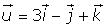and, find the productand verify that this vector is orthogonal toand. Also, find the vectorand compare it with.

## Exercise 4

Consider the following figure:Determine:

1 The coordinates of D if ABCD is a parallelogram.

## Exercise 5

Given the points A = (1, 0, 1), B = (1, 1, 1) and C = (1, 6, a), determine:

1 What values of a are collinear.

2 Determine if values exist for a so that A, B and C are three vertices of a parallelogram of area 3. If values do exist, determine the coordinates of C:

## Exercise 6

A = (−3, 4, 0), B = (3, 6, 3) and C = (−1, 2, 1) are the three vertices of a triangle.

1. Calculate the cosine of each of the three angles in the triangle.

2. Calculate the area of the triangle.

## Solution of exercise 1

Find two unit vectors for (2, −2, 3) and (3, −3, 2) and determine the orthogonal vector for the two.## Solution of exercise 2

Find a unit vector that is perpendicular toand.## Solution of exercise 3

Given the vectorsand, find the productand verify that this vector is orthogonal toand. Also, find the vectorand compare it with.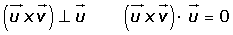## Solution of exercise 4

Consider the following figure:Determine:

1 The coordinates of D if ABCD is a parallelogram.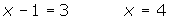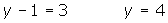## Solution of exercise 5

Given the points A = (1, 0, 1), B = (1, 1, 1) and C = (1, 6, a), determine:

1 What values of a are collinear.

If A, B and C are collinear, the vectorsandare linearly dependent and have proportional components.2 Determine if values exist for a so that A, B and C are three vertices of a parallelogram of area 3. If values do exist, determine the coordinates of C: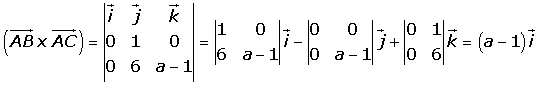## Solution of exercise 6

A = (−3, 4, 0), B = (3, 6, 3) and C = (−1, 2, 1) are the three vertices of a triangle.

1. Calculate the cosine of each of the three angles in the triangle.2. Calculate the area of the triangle.Did you like the article?(1 votes, average: 5.00 out of 5)Loading...

Emma

I am passionate about travelling and currently live and work in Paris. I like to spend my time reading, gardening, running, learning languages and exploring new places.

Did you like
this resource?

Bravo!